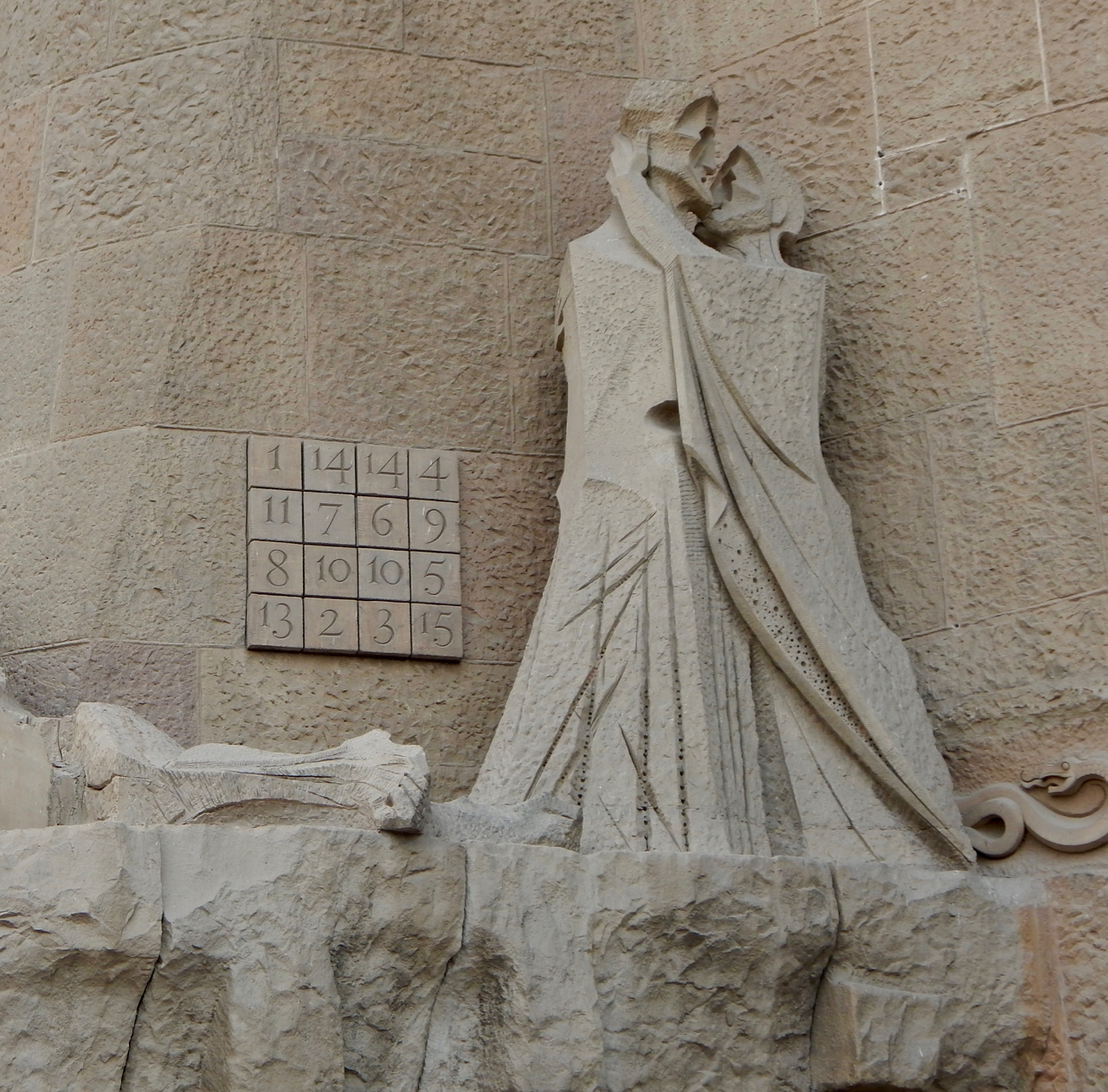# Jesus’s Magic Square(Click to enlarge)

From an exterior wall of the Sagrada Familia Church designed by Antoni Gaudi.

Barcelona, Spain

The sum of the numbers in every row is 33.

The sum of the numbers in every column is 33.

The sum of the numbers in each diagonal is 33.

The sum of the numbers in the four corners is 33.

The sum of the numbers in the center 2×2 square is 33.

The sum of the numbers in each 2×2 square in each of the corners is 33.